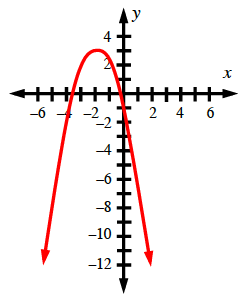### Home > CCAA8 > Chapter 10 Unit 11 > Lesson CCA: 10.3.1 > Problem10-112

10-112.

For the function $f(x)=−(x+2)^2+3$:

1. Use your graphing short cuts to graph the function.1. Where is the maximum value found on this function?

What do you call the highest or lowest point on a parabola?

1. What is this maximum value?

The maximum value is the greatest $y$-value.